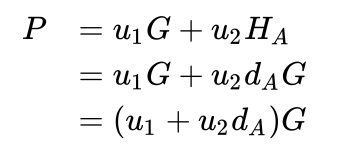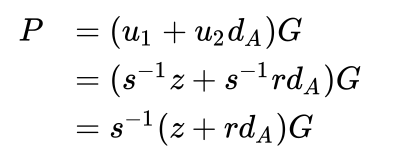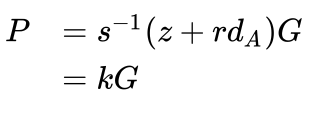0%

# RSA（模幂运算）

## 算法描述

RSA算法的具体描述如下：

（1）任意选取两个不同的大素数p和q计算乘积$n=pq, φ(n)=(p-1)(q-1);$

n的二进制长度是密钥长度。实际应用中，RSA密钥一般是1024位，重要场合则为2048位。

（2）任意选取一个大整数e，满足 gcd(e,φ(n)) = 11< e < φ(n)，整数e用做加密钥（注意：e的选取是很容易的，例如，所有大于p和q的素数都可用）；

（3）确定的解密钥d，满足 (de)mod φ(n) = 1 ，即de = kφ(n) +1, k≥1是一个任意的整数；所以，若知道e和φ(n)，则很容易计算出d；

（4）公开整数n和e，秘密保存d ；

（5）将明文m（m<n是一个整数）加密成密文c，加密算法为$c=E(m)=m^emodn$ , n和e为公钥；

（6）将密文c解密为明文m，解密算法为$m=D(c)=c^dmodn$，n和d为私钥；

## RSA签名验证

RSA密码体制既可以用于加密又可以用于数字签名。下面介绍RSA数字签名的功能。

• 1.对于消息m签名为：sign ≡ m ^d mod n
• 2.验证：对于消息签名对（m，sign），如果m ≡ sign ^e mod n，则sign是m的有效签名

(((c^e)%n)^d)%n =(c^(d*e))%n =(c^(k*t+1))%n =(c*((c^k)^t))%n =((c%n)*(((c^k)^t)%n))%n =((c%n)*(((c^k)%n)^t)%n)%n

ϕ(n)是欧拉函数，ϕ(n)=从1到n-1中所有与n互质整数的个数。k=ϕ(n)。根据欧拉定理(1)，对于任意c，如果c与n互质，那么：(c^k)%n=(c^ϕ(n))%n=1

(((c^e)%n)^d)%n=c

# ECC（几何离散）

1. 使用Schoof的算法去计算椭圆曲线的阶 N 。
2. 找到 N 所有的因子。
3. 对于 N 的每一个因子 n ,计算 nP 。
4. 找到最小的且满足 nP=0 的 n , n 就是子群的阶。

NP=n(hP)=0 , 令G=hP => nG=0

## 找基点

1. 计算椭圆曲线的阶 N 。
2. 选择一个阶为 n 的子群。n必须是素数且必须是 N 的因子。
3. 计算辅因子 h = N / n 。
4. 在曲线上选择一个随机的点 P 。
5. 计算 G = hP 。
6. 如果 G 是0，那么回到步骤4。否则我们就已经找到了阶为 n 和辅因子是 h 的子群的基点。

## ECDH

1. Alice 和 Bob 生成各自的私钥和公钥，Alice 的私钥为 $d_A$ ，公钥为 $H_A = d_AG$ 。Bob 的私钥为 $d_B$，公钥为 $H_B = d_BG$ ，注意，Alice 和 Bob 需要使用一样的主要参数：在同一条曲线的同一个有限域上选择一样的基点 G。
2. Alice 和 Bob 通过不安全信道交换各自的公钥$H_A$和 $H_B$ ，中间人可以窃听到 $H_A$和 $H_B$，但是在无法攻破离散对数难题的情况下无法得到 $d_A$ 和 $d_B$ 。
3. Alice 计算 $S=d_AH_B$ （使用自身的私钥和 Bob 的公钥），Bob 计算 $S=d_BH_A$ （使用自身的私钥和 Alice 的公钥），双方求得的 S 是一样的，因为 $S=d_AH_B=d_A(d_BG)=d_B(d_AG)=d_BH_A$

## ECDSA

Alice 想要使用她的私钥 $d_A$ 来签名，Bob 想用 Alice 的公钥 $H_A$ 要验证签名，只有 Alice 才能提供正确的签名，而每个人都可以验证签名。

Alice 使用算法来签名的步骤如下：

1. 在 {1,…, n-1} 范围内选取一个随机数 k （ n 是子群的阶）
2. 计算点 P=kG（ G 是子群的基点）
3. 计算数字 $r = x_p[mod]n$ （ $x_p$是p的x轴坐标）
4. 如果 r=0 ，另选一个 k 并重新计算
5. 计算 $s=k^{-1}(z+rd_A)[mod]n$ （ $d_A$是 Alice 的私钥， $k^{-1}$是 k mod n 的乘法逆元）
6. 如果 s=0 ，另选一个 k 并重新计算

(r, s) 就是签名。

### 验证签名

1. 计算整数 $u_1 = s^{-1}z [mod] n$
2. 计算整数 $u_2 = s^{-1}r[mod]n$
3. 计算点 $P=u_1G + u_2H_A$

### 算法的正确性# Schnorr

• G：椭圆曲线基点。
• m：待签名的数据，通常是一个32字节的哈希值。
• x：私钥。P = xG，P为x对应的公钥。
• H()：哈希函数。
• 示例：写法H(m || R || P)可理解为：将m, R, P三个字段拼接在一起然后再做哈希运算。

## 生成签名

1. 选择一个随机数k, 令 R = k*G
2. s = k + H(m || R || P)*x

## 验证签名

s*G = R + H(m || R || P)P

1. s值的定义：s = k + H(m || R || P)*x，等式两边都乘以椭圆曲线G，则有：
2. sG = kG + H(m || R || P)*x*G，又因R = kG, P = xG，则有：
3. sG = R + H(m || R || P)P，椭圆曲线无法进行除法运算，所以第3步的等式，无法向前反推出第1步，就不会暴露k值以及x私钥。同时，也完成了等式验证。

## 组签, Group Signature

• 椭圆曲线基点：G
• 待签名的数据：m
• 哈希函数：H()
• 私钥：x1，x2，公钥：P1=x1G, P2=x2G
• 随机数：k1, k2，并有 R1=k1G, R2=k2G
• 组公钥：P = P1 + P2

• 私钥x1和x2的签名为：(R1, s1), (R2, s2)。
• 两个签名相加得到组签名：(R, s)。其中：R = R1 + R2, s = s1 + s2。

### 推演过程：

1. R = R1 + R2, s = s1 + s2

2. 已知：s1 = k1 + H(m || R || P)*x1s2 = k2 + H(m || R || P)*x2

3. 两边同时乘以G，则有：

4. 完成证明，并从两个合作方推演至N个合作方

## 多签

m-of-n多重签名使用默克尔树；

# BLS

Boneh-Lynn-Shacham

Schnorr 签名方案是非常了不起的，如果我们做得对，我们可以将交易中的所有签名和公钥组合为一个密钥和一个签名，没有人会发现它们对应于多个密钥。区块验证也可以变得更快，因为我们可一次性验证所有签名。但它也存在着一些问题：

1. 多重签名方案需要多轮通信，这会使冷存储变得非常烦人；
2. 对于签名聚合，我们必须依赖随机数生成器，我们不能像在 ECDSA 中那样确定地选择随机点 R；
3. m-of-n 多重签名方案是棘手的，我们需要制作一个公共密钥的默克尔树（merkle tree），对于大数的 m 和 n 来说，这颗默克尔树可以变得相当大；
4. 我们不能将区块中的所有签名组合为单个签名；

BLS 签名可修复上述所有问题，我们不需要随机数，区块中的所有签名都可以组合成单个签名，m-of-n 类型的多重签名非常简单，我们不需要签名者之间进行多轮通信。此外，BLS 签名相比 Schnorr 签名或 ECDSA 签名要小 2 倍，其签名不是一对，而是一个单曲线点。

S = a1×S1+a2×S2+a3×S3

P = a1×P1+a2×P2+a3×P3

ai = hash(Pi, {P1,P2,P3})

## m-of-n签名

P = a1×P1+a2×P2+a3×P3, ai = hash(Pi, {P1,P2,P3})

MKi = (a1⋅pk1)×H(P, i)+(a2⋅pk2)×H(P, i)+(a3⋅pk3)×H(P, i)

e(G, MKi)=e(P, H(P,i))

## 签名

S1 = pk1×H(P, m)+MK1, S3=pk3×H(P, m)+MK3

(S’, P’) = (S1+S3, P1+P3)

e(G, S’) = e(P’, H(P, m))⋅e(P, H(P, 1)+H(P, 3))

e(G, S’) = e(G, S1+S3)=e(G, pk1×H(P, m)+pk3×H(P, m)+MK1+MK3) =e(G, pk1×H(P,m)+pk3×H(P, m))⋅e(G, MK1+MK3)=e(pk1×G+pk3×G, H(P, m))⋅e(P, H(P, 1)+H(P,3))=e(P’, H(P, m))⋅e(P, H(P, 1)+H(P, 3))

BLS 签名的弊端：配对效率低下。